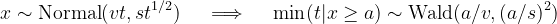﻿WaldDistribution Class# WaldDistribution Class

Represents a Wald (Inverse Gaussian) distribution.Inheritance Hierarchy

Namespace:  Meta.Numerics.Statistics.Distributions
Assembly:  Meta.Numerics (in Meta.Numerics.dll) Version: 4.1.4Syntax
`public sealed class WaldDistribution : ContinuousDistribution`

The WaldDistribution type exposes the following members.Constructors
NameDescriptionWaldDistribution
Initializes a new Wald distribution.
TopProperties
NameDescriptionExcessKurtosis
Gets the excess kurtosis of the distribution.
(Overrides UnivariateDistributionExcessKurtosis.)Mean
Gets the mean of the distribution.
(Overrides UnivariateDistributionMean.)Median
Gets the median of the distribution.
(Inherited from ContinuousDistribution.)Shape
Gets the shape parameter of the distribution.Skewness
Gets the skewness of the distribution.
(Overrides UnivariateDistributionSkewness.)StandardDeviation
Gets the standard deviation of the distribution.
(Inherited from UnivariateDistribution.)Support
Gets the interval over which the distribution is non-vanishing.
(Overrides ContinuousDistributionSupport.)Variance
Gets the variance of the distribution.
(Overrides UnivariateDistributionVariance.)
TopMethods
NameDescriptionCentralMoment
Computes a central moment of the distribution.
(Overrides ContinuousDistributionCentralMoment(Int32).)Cumulant
Computes a cumulant of the distribution.
(Overrides UnivariateDistributionCumulant(Int32).)Equals
Determines whether the specified object is equal to the current object.
(Inherited from Object.)ExpectationValue
Computes the expectation value of the given function.
(Inherited from ContinuousDistribution.)FitToSample
Determines the parameters of the Wald distribution that best fits a sample.GetHashCode
Serves as the default hash function.
(Inherited from Object.)GetRandomValue
Generates a random variate.
(Overrides ContinuousDistributionGetRandomValue(Random).)GetRandomValues
Generates the given number of random variates.
(Inherited from ContinuousDistribution.)GetType
Gets the Type of the current instance.
(Inherited from Object.)Hazard
Computes the hazard function.
(Inherited from ContinuousDistribution.)InverseLeftProbability
Returns the point at which the cumulative distribution function attains a given value.
(Inherited from ContinuousDistribution.)InverseRightProbability
Returns the point at which the right probability function attains the given value.
(Inherited from ContinuousDistribution.)LeftProbability
Returns the cumulative probability to the left of (below) the given point.
(Overrides ContinuousDistributionLeftProbability(Double).)ProbabilityDensity
Returns the probability density at the given point.
(Overrides ContinuousDistributionProbabilityDensity(Double).)RawMoment
Computes a raw moment of the distribution.
(Overrides ContinuousDistributionRawMoment(Int32).)RightProbability
Returns the cumulative probability to the right of (above) the given point.
(Overrides ContinuousDistributionRightProbability(Double).)ToString
Returns a string that represents the current object.
(Inherited from Object.)
TopRemarks

The Wald distribution, also called the inverse Gaussian distribution, is the distribution of first passage times for Brownian motion.

In Brownian motion, a particle moves randomly so that its position at any given time is distributed normally with a mean that increases linearly and a standard deviation that increases with the square root of time. The first passage time is the earliest time that its position reaches a given level. This first passage time is Wald distributed with mean and shape parameters related to the drift, noise, and threshold.This may appear a very obscure an technical relationship, but it turns out to have myriad applications: to stock prices, ballot counting, neurological response times, and earthquake prediction.See Also